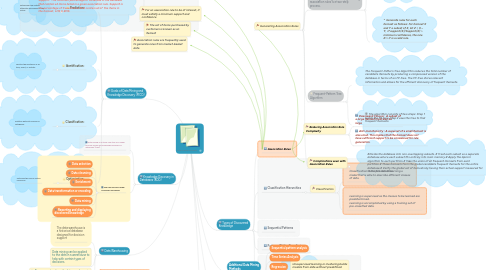# Data Maining concepts

Organize and structure your thoughts to write an essay

Get Started. It's FreeData Maining concepts by## 1. Goals of Data Mining and Knowledge Discovery (PICO)

### 1.1. Prediction:

1.1.1. Determine how certain attributes will behave in the future

### 1.2. Identification:

1.2.1. Identify the existence of an item, event, or activity.

### 1.3. Classification:

1.3.1. Partition data into classes or categories.

### 1.4. Optimization:

1.4.1. Optimize the use of limited resources

## 2. Knowledge Discovery in Databases (KDD)

### 2.2. The KDD process model comprises six phases

2.2.1. Data selection

2.2.2. Data cleansing

2.2.3. Enrichment

2.2.4. Data transformation or encoding

2.2.5. Data mining

2.2.6. Reporting and displaying discovered knowledge

## 3. Data Warehousing

### 3.3. Proper construction of a data warehouse is fundamental to the successful use of data mining

3.3.1. Find examples

3.3.2. Find relevant quotes

3.3.3. References

3.3.3.1. Books

3.3.3.2. News sources

3.3.3.3. Blogs

3.3.3.4. Supporting Data

3.3.3.4.1. Expert reports

3.3.3.4.2. Third party research

3.3.3.4.3. Survey data

3.3.3.4.4. Size of topic

## 9. Types of Discovered Knowledge

### 9.2. Classification Hierarchies

9.2.1. Classification

9.2.1.1. Classification is the process of learning a model that is able to describe different classes of data.

9.2.1.2. Learning is supervised as the classes to be learned are predetermined. Learning is accomplished by using a training set of pre-classified data.

### 9.5. Clustering

9.5.1. Unsupervised learning or clustering builds models from data without predefined classes.

9.5.2. The goal is to place records into groups where the records in a group are highly similar to each other and dissimilar to records in other groups.

9.5.3. The k-Means algorithm is a simple yet effective clustering technique.

## 10. Generating Association Rules

### 10.1. The Apriori Algorithm

10.1.1. The Apriori algorithm was the first algorithm used to generate association rules. The Apriori algorithm uses the general algorithm for creating association rules together with downward closure and anti-monotonicity.

### 10.2. The Sampling Algorithm

10.2.1. The sampling algorithm selects samples from the database of transactions that individually fit into memory. Frequent itemsets are then formed for each sample.

### 10.3. The general algorithm for generating association rules is a two-step process.

10.3.1. * Generate all itemsets that have a support exceeding the given threshold. Itemsets with this property are called large or frequent itemsets.

10.3.2. * Generate rules for each itemset as follows: For itemset X and Y a subset of X, let Z = X – Y; If support(X)/Support(Z) > minimum confidence, the rule Z=>Y is a valid rule.

### 10.4. Frequent-Pattern Tree Algorithm

10.4.1. The Frequent-Pattern Tree Algorithm reduces the total number of candidate itemsets by producing a compressed version of the database in terms of an FP-tree. The FP-tree stores relevant information and allows for the efficient discovery of frequent itemsets.

10.4.2. The algorithm consists of two steps: Step 1 builds the FP-tree. Step 2 uses the tree to find frequent itemsets.

### 10.5. The Partition Algorithm

10.5.1. #Divide the database into non-overlapping subsets. # Treat each subset as a separate database where each subset fits entirely into main memory.# Apply the Apriori algorithm to each partition.# Take the union of all frequent itemsets from each partition.# These itemsets form the global candidate frequent itemsets for the entire database.# Verify the global set of itemsets by having their actual support measured for the entire database.